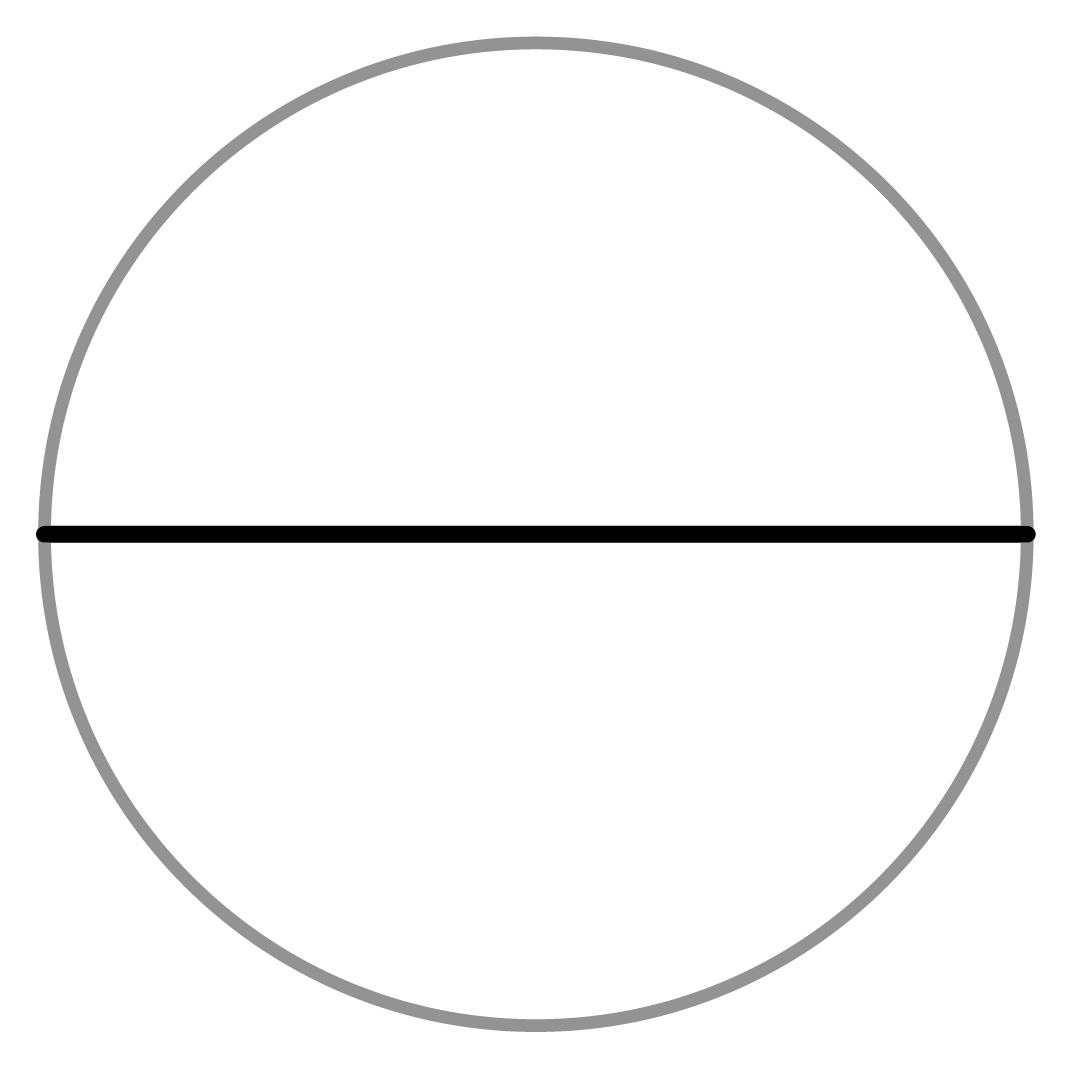Chapter overviewMaths

Number and place value

Multiplication and division

Fractions

Measurement

Geometry - properties of shapes

Statistics

Maths

# 2D shapes: Types and properties0%

Summary

# 2D shapes: Types and properties

## In a nutshell

2D shapes are flat shapes that are sometimes called plane shapes. There are many different types of 2D shapes. Regular polygons have equal side lengths and equal angles.

## Polygons

Polygons are straight-sided shapes. Regular polygons have sides of equal length, and equal angles. Irregular polygons have at least one side or angle that is different in size. Below is a table that shows the names of different shapes depending on how many sides they have:

#### Diagram

Triangle

$3$​​

$4$​​

Pentagon
$5$​​

Hexagon
$6$​​

Heptagon
$7$​​
Octagon
$8$​​

Quadrilaterals are four-sided shapes. There are a number of different types of quadrilateral:

#### diagram

Square

• Four sides of equal length.
• Four right angles.
• The diagonals meet at right angles.
• Two pairs of parallel sides.
Rectangle
• Two pairs of equal sides.
• Four right angles.
• Two pairs of parallel sides.
Rhombus
• Four sides of equal length.
• Opposite sides are parallel.
• Opposite angles are equal.
Parallelogram
• Opposite sides are the same length.
• Opposite sides are parallel.
• Opposite angles are equal.
Trapezium
• One pair of parallel sides.
Kite
• Two pairs of equal sides next to each other.
• No sides are parallel.

## Triangles

A triangle is a 2D plane shape with three straight sides and three angles. There are four types of triangle:

#### diagram

Equilateral triangle
• All three sides are equal.
• All three angles are equal.
Isosceles triangle
• Two sides are equal.
• Two angles are equal.

Scalene triangle
• ​All sides are different.
• All angles are different.

Right-angled triangle
• One angle is a right angle.

## Circles

A circle is a shape made up of all points in a plane that are a fixed distance from the centre. There are a number of properties of a circle:

• Radius - the distance from the edge to the centre of a circle.
• Diameter - the distance across a circle through its centre.
• Circumference - the length of the outside edge of a circle.

Note: The diameter of a circle is twice the radius.FAQs

• Question: What is the circumference of a circle?

Answer: The circumference is the length of the outside edge of a circle.

• Question: What is a triangle?

Answer: A triangle is a 2D plane shape with three straight sides and three angles.

• Question: What is a circle?

Answer: A circle is a shape made up of all points in a plane that are a fixed distance from the centre.

• Question: What is a polygon?

Answer: A polygon is a straight-sided 2D (plane) shape.

• Question: What is the name for a four-sided shape?